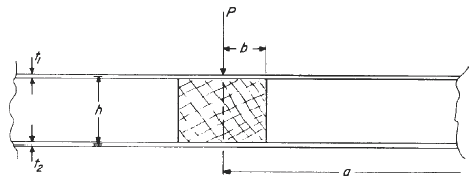Related Resources: Engineering Materials

### Bending of Circular Sandwich Plate by Load Applied Through an Insert

Structural Beam Deflection and Stress Formula & Deflection Calculator
Flat Plates Stress, Deflection Equations and Calculators

Bending of a Circular Sandwich Plate by Load Applied Through an Insert

ABSTRACT
An analytical solution is presented for the bending of a circular sandwich plate by a normal load applied through a rigid clamped insert. The facings are assumed to be isotropic, while the core is assumed to possess negligible in-plane stiffnesses and a single transverse modulus of rigidity. During bending, the two facings have identical curvatures and the core shear stress is constant throughout the thickness
of the core.

Introduction

This research extends the theory of Ericksen3 for symmetrical bending of a circular sandwich plate to the case of bending by a point load applied through a rigid, clamped circular insert (or hub) at the center of the plate. The analysis is intended to provide guidance toward the design of intersandwich panel connectors.

Solutions are obtained for plates clamped and simply supported at their outer edges. The system is pictured in figure 1, showing the exterior plate and its interior sect ion. The facings of the plate are composed of isotropic materials, while the core material is assumed to possess negligible in-plane stiffnesses. Shear deflections

of the facings and effects due to bending of the core are neglected. As is usually assumed with small deflection bending of rectangular sandwich plates, the shear stress in the core is taken to be constant throughout the core thickness. Moreover, the facings are assumed to have identical curvatures during bending. The theory and basic equations herein generalize those of Ericksen by allowingthe sandwich top and bottom facings to be composed of either the same or different materials which have the same Poisson’s ratio. Analytical solutions are obtained for plate bending and shear deflections, core shear stress, and facing bending stresses corresponding to each plate outer edge condition. Normalized values of these quantities are presented in a series of design curves.Related: